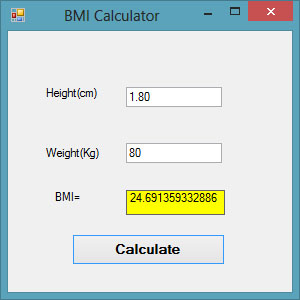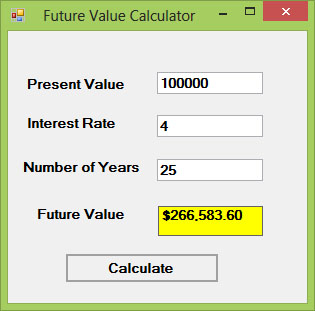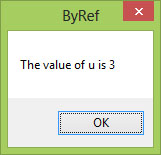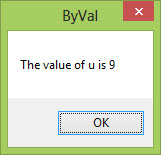# Visual Basic 2010 Lesson 12- Introduction to Functions

#### [Lesson 11] << [CONTENTS] >> [Lesson 13]

A Visual Basic 2010 function is a type of procedure that returns a value which is passed on to the main procedure to finish the execution. A function is similar to a sub procedure but there is one major difference, a function returns a value whilst a sub procedure does not.

In Visual Basic 2010, there are two types of functions, the built-in functions and the functions created by the programmers. Functions created by the programmer are also known as user-defined functions. In this lesson, we shall learn how to create the user-defined function.

### 12.1 Creating User-Defined Functions

To create a user- defined function in Visual Basic 2010, you can use the following syntaxes:

Public Function functionName (Argument As dataType,……….) As dataType
or
Private Function functionName (Argument As dataType,……….) As dataType

The keyword Public indicates that the function is applicable to the whole project and the keyword Private indicates that the function is only applicable to a certain module or procedure. The argument is a parameter that can pass a value back to the function.There is no limit to the number of arguments you can put in.

#### Example 12.1: BMI Calculator

This BMI calculator is a Visual Basic 2010 program that can calculate the body mass index of a person based on his or her body weight in kilogram and the body height in meter. BMI can be calculated using the formula weight/( height )2, where weight is measured in kg and height in meter.

If the  BMI is more than 30, a person is considered obese. You can refer to the following range of BMI values for your weight status.

• Underweight = <18.5
• Normal weight = 18.5-24.9
• Overweight = 25-29.9
• Obesity = BMI of 30 or greater

The Code

```Public Class Form1

Private Function BMI(Height As Single, weight As Single) As Double
BMI = weight / Height ^ 2
End Function

Private Sub BtnCal_Click(sender As Object, e As EventArgs) Handles BtnCal.Click

Dim h As Single, w As Single
h = Val(TextBox1.Text)
w = Val(TextBox2.Text)
LblBMI.Text = BMI(h, w)
End Sub
End Class
```

#### The outputFigure 12.1

#### Example 12.2: Future Value Calculator

The concept of future value is related to time value of money. If you deposit your money in a bank as a savings account or a fixed deposit account for a certain period of time, you will earn a certain amount of money based on the compound interest computed periodically, and this amount is added to the principal if you continue to keep the money in the bank. Interest for the following period is computed based on the initial principal plus the interest, this amount becomes the new principal. Subsequent interests are computed in the same manner.

For example, let’s say you deposit \$1000 in a bank and the bank is paying you 5% compound interest annually. After the first year, you will earn an interest of \$1000×0.05=\$50. The new principal will be \$1000+\$1000×0.05=\$1000(1+0.05)=\$1000(1.05)=\$1050. After the second year, the new principal is \$1000(1.05)x1.05=\$1000(1.05)2 =\$1102.50. This new principal is called the future value.

Following the above calculation, the future value after n years will be

`FV = PV * (1 + i / 100)n`

Where PV represents the present value, FV represents the future value, i is the interest rate and n is the number of periods (Normally months or years).

The Code

```Public Class Form1
Private Function FV(pv As Single, i As Single, n As Integer) As Double
FV = pv * (1 + i / 100) ^ n
End Function

Private Sub BtnCal_Click(sender As Object, e As EventArgs) Handles BtnCal.Click

Dim FutureVal As Single
Dim PresentVal As Single
Dim interest As Single
Dim period As Integer
PresentVal = TxtPV.Text

interest = TxtInt.Text
period = TxtN.Text

FutureVal = FV(PresentVal, interest, period)
LblFV.Text = Format(FutureVal, "\$#,##0.00")
End Sub
End Class
```

The OutputFigure 12.2

#### 12.2 Passing Arguments by Value and by Reference

Functions can be called by value or called by reference.  By default, the arguments in the function are passed by reference. If arguments are passed by reference, original data will be modified and no longer preserved. On the one hand, if arguments are passed by value, original data will be preserved. The keyword to pass arguments by reference is ByRef and the keyword to pass arguments by value is ByVal.

For example,

`Private Function FV(ByVal pv As Single, ByRef i As Single, n As Integer) As Double`

The function FV receives pv by value, i by reference and n by reference. Notice that although ByRef is not used to pass n, by default it is passed by reference.

##### Example 12.2(a)

In this example, we created two functions that compute the square root of a number, the first uses the keyword ByRef and the second uses the keyword ByVal.

The Code

```Public Class Form1

Private Function sqroot(ByRef x As Single) As Double
x = x ^ 0.5
sqroot = x
End Function

Private Function sqroot1(ByVal y As Single) As Double
y = y ^ 0.5
sqroot1 = y
End Function

Private Sub Button1_Click(sender As Object, e As EventArgs) Handles Button1.Click
Dim u As Single
u = 9
MsgBox(3 * sqroot(u), , "ByRef")
MsgBox("Value of u is " & u, , "ByRef")

End Sub

Private Sub Button2_Click(sender As Object, e As EventArgs) Handles Button2.Click
Dim u As Single
u = 9
MsgBox(3 * sqroot1(u), , "ByVal")
MsgBox("Value of u is " & u, , "ByVal")
End Sub

End Class
```

#### The Output

###### Case 1: Passing argument using ByRefFigure 12.3

Notice that the value of u has been changed to 3

###### Case 2: Passing argument using ByValFigure 12.4

Notice that the value of u remains unchanged.